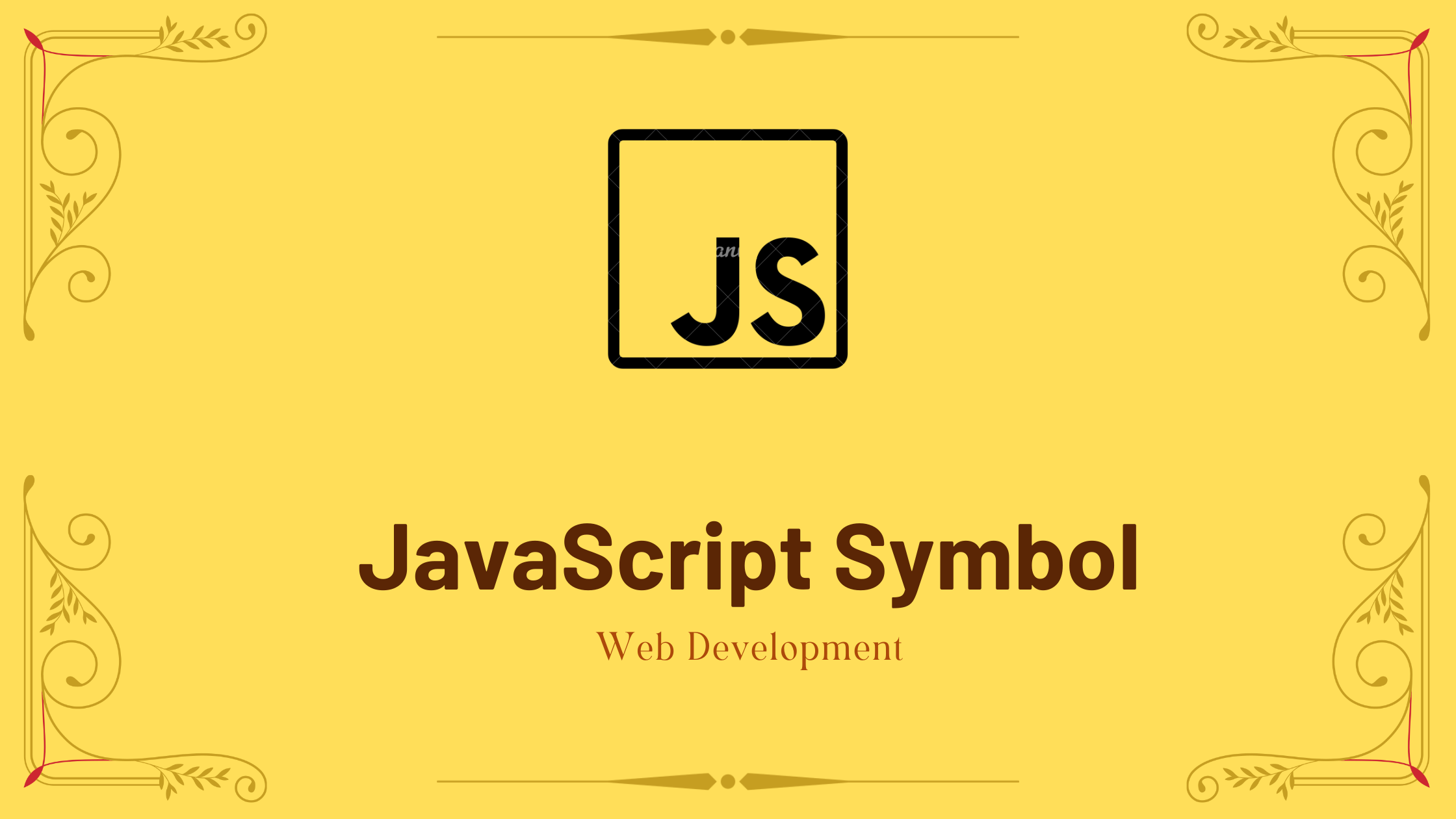## JavaScript Symbol with Example

In this tutorial, you will learn about JavaScript symbol with the help of examples.

Symbols are a new primitive class in ES6. Symbols are one-of-a-kind identifiers. They can be created using the factory function Symbol(), which returns a Symbol, just like their primitive counterparts (Number, String, and Boolean). Every time you invoke the factory function, a new and distinct symbol is generated.

A symbol is a new primitive data type introduced in JavaScript ES6. Symbols are immutable (they cannot be modified) and unique. As an example:

```// two symbols with the same description

const value1 = Symbol('hello');
const value2 = Symbol('hello');

console.log(value1 === value2); // false```

Though value1 and value2 both contain the same description, they are different.

Contents

## Creating Symbol

To create a Symbol, use the Symbol() function. As an example:

```// creating symbol
const x = Symbol()

typeof x; // symbol```

As its description, you can provide an optional string. As an example:

```const x = Symbol('hey');
console.log(x); // Symbol(hey)```

## Access Symbol Description

The. operator is used to get the description of a symbol. As an example:

```const x = Symbol('hey');
console.log(x.description); // hey```

## Add Symbol as an Object Key

Using square brackets [], you can insert symbols as a key in an object. As an example:

```let id = Symbol("id");

let person = {
name: "Jack",

// adding symbol as a key
[id]: 123 // not "id": 123
};

console.log(person); // {name: "Jack", Symbol(id): 123}```

## Symbols are not included in for…in Loop

Symbolic characteristics are not iterated over in the for…in loop. As an example:

```let id = Symbol("id");

let person = {
name: "Jack",
age: 25,
[id]: 12
};

// using for...in
for (let key in person) {
console.log(key);
}```

Output

name
age

## Benefit of Using Symbols in Object

If the same code snippet is used in multiple apps, utilise Symbols in the object key. Because you may avoid redundancy by using the same key name in different codes. As an example:

```let person = {
name: "Jack"
};

// creating Symbol
let id = Symbol("id");

// adding symbol as a key
person[id] = 12;```

If the person object in the preceding programme is also utilised by another programme, you wouldn’t want to add a property that another programme could access or edit. As a result, by utilising Symbol, you generate a one-of-a-kind property that you may use.

If the other programme also wants to utilise a property named id, simply create a Symbol named id to avoid duplication. As an example:

```let person = {
name: "Jack"
};

let id = Symbol("id");

person[id] = "Another value";```

Even if the same name is used to store values in the preceding program, the Symbol data type will have a unique value.

If the string key had been used in the preceding program, the later programme would have changed the value of the property. As an example:

```let person = {
name: "Jack"
};

// using string as key
person.id = 12;
console.log(person.id); // 12

// Another program overwrites value
person.id = 'Another value';
console.log(person.id); // Another value```

In the preceding program, the second user.id replaces the previous value.

## Symbol Methods

There are various methods available with Symbol.

### Example: Symbol Methods

```// get symbol by name
let sym = Symbol.for('hello');
let sym1 = Symbol.for('id');

// get name by symbol
console.log( Symbol.keyFor(sym) ); // hello
console.log( Symbol.keyFor(sym1) ); // id```

## Symbol Properties

### Example: Symbol Properties Example

```const x = Symbol('hey');

// description property
console.log(x.description); // hey

const stringArray = ['a', 'b', 'c'];
const numberArray = [1, 2, 3];